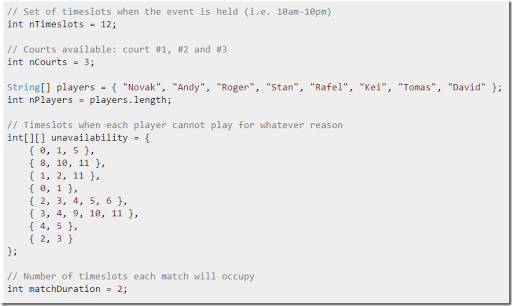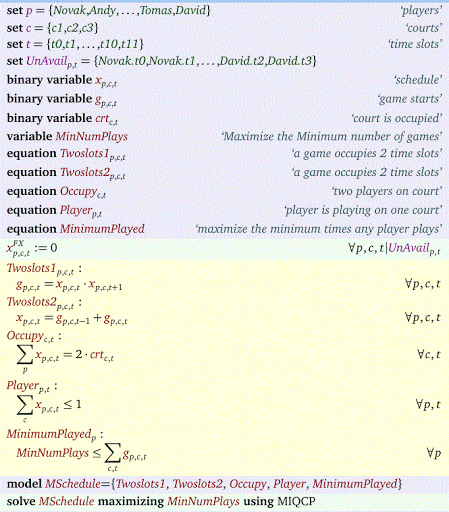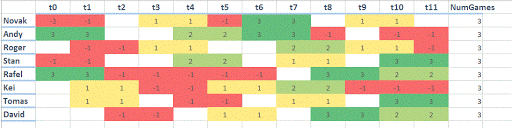## Thursday, March 3, 2016

### Tennis Scheduling

Scheduling models are not always simple to write down. Here someone is quite stuck in the mud trying to implement a scheduling model in the Constraint Programming system Choco. Let’s see if some Math Programming can do the job.

This is what we know:So:

• We have 12 time periods
• We have three courts
• We have 8 players
• We have some data about forbidden assignments of players to slots
• A game uses two (consecutive) time slots

Here is my MIQCP model:It has one non-linear (quadratic) equation: Twoslots1. However this is a binary multiplication, so we can easily linearize this using the familiar construct:

 \begin{align}&g_{p,c,t} \le x_{p,c,t}\\ &g_{p,c,t} \le x_{p,c,t+1}\\&g_{p,c,t} \ge x_{p,c,t}+x_{p,c,t+1}-1 \end{align}

Now we have a straight MIP model. We try to prevent someone playing very few games, so we maximize the minimum number of games a person plays. The final results look like:The red cells indicate: not allowed. Otherwise the numbers in the cells represent the tennis-court.